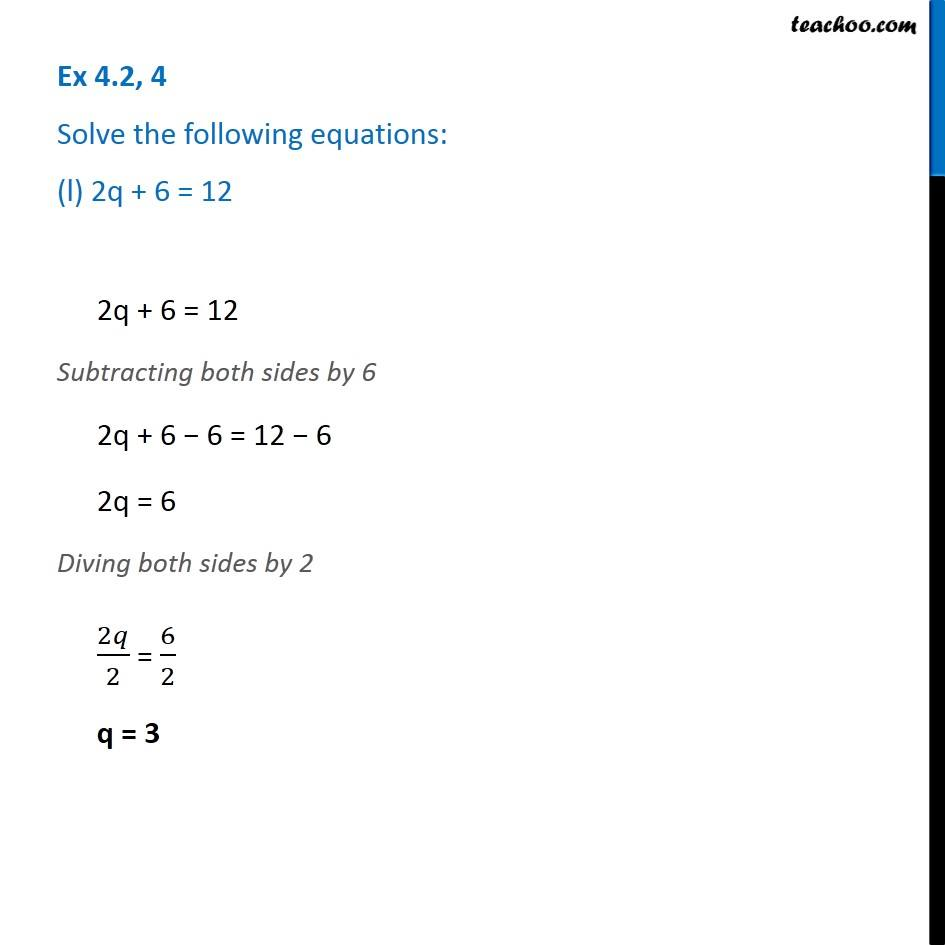Ex 4.2

Chapter 4 Class 7 Simple Equations
Serial order wiseLearn in your speed, with individual attention - Teachoo Maths 1-on-1 Class

### Transcript

Ex 4.2, 4 Solve the following equations: (l) 2q + 6 = 122q + 6 = 12 Subtracting both sides by 6 2q + 6 − 6 = 12 − 6 2q = 6 Diving both sides by 2 2𝑞/2 = 6/2 q = 3